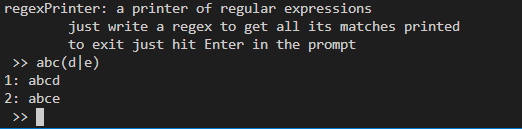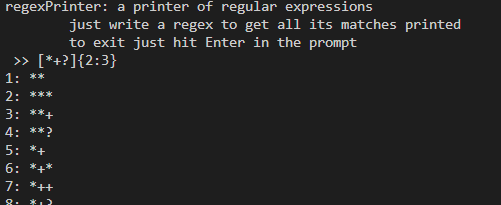## Finding all strings a regular expression can find

A regular expression, without much rigor, is a very compact way of representing several different strings. Given a regular expression (regex), can I find out all the strings the regex can find?

One common use of regular expressions is to look for strings that have a certain structure in a bigger string (say a text). As an example, the regular expression abc(d|e) can be used to look for the strings "abcd" and "abce", where the character | denotes we have to make a choice (see image above). Thus cat|dog would match the strings "cat" and "dog". There are other special symbols that have meanings and purposes.One very interesting question that arises is: given a regular expression, what are the strings matched by it? To answer that question I wrote a small Python program, that I called regexPrinter, that prints all strings matched by a given regular expression! In order to manage that task, I chose a subset of the regex syntax that I wanted to be able to print and also decided that whenever a piece of a pattern was infinite, at a given point the program would just print "..." to denote that infinity. This way, for any regex given, the program always stops.

The regexPrinter supports:

• the * operator, that denotes that $$0$$ or more repetitions are to be matched. For example, ah* matches "a", "ah", "ahh", ...;
• the + operator that denotes that $$1$$ or more repetitions are to be matched. For example, (hue)+ matches "hue", "huehue", "huehuehue", ...;
• the ? operator that denotes either $$0$$ or $$1$$ occurrences of the preceding pattern. For example, woo(hoo)? matches "woo" and "woohoo";
• the {a:b} operator that matches no less than $$a$$ and no more than $$b$$ repetitions of the preceding pattern. As an example, su{1:3}re matches the strings "sure", "suure" and "suuure";
• the | operator that denotes a choice. cat|kat matches "cat" and "kat" and thank(s| you) matches "thanks" and "thank you";
• the [] denote that only one pattern from the ones given are to be matched. For example [abc] matches "a", "b" and "c";
• the parenthesis () that are used to group things. One thing to note is that the quantifiers *+?{:} all have higher precedence than string concatenation e.g., ab? is interpreted as a(b?) and not (ab)?.

Please bear in mind that any character with no special meaning is interpreted literally, except inside the grouping operator [], where every character is interpreted literally. That is, [ab*] matches "a", "b" and "*", while b&=' will match the string "b&='".The code for the program can be found here on GitHub and all it takes is vanilla Python 3 to run. Just run the script and you will be prompted to insert regular expressions. The techniques I used were very, very similar to the ones I used to create my toy programming language, as I saw in this blog series. The way I went about writing this program was by implementing a parser for a subset of the grammar used by regular expressions, generating a tree representation for the regex and then visiting all nodes of the tree, where each node knows how to print itself.